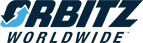Print Page  Close Window

News Releases

 Get News Alerts by E-mailYear: Select Year");");");");");");");");");");");");");");");");");");");");");");");");");");");");");");");");");");");");");");");");");");");");");");");");");");");");");");");");");");");");");");");");");");");");");");");");");");");");");");");");");");");");");");");");");");");");");");");");");");");");");");");");");");");");");");");");");");");");");");");");");");");");
Category:There are currently no news releases.